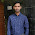## Tuesday, October 16, 2012

### Basic Electronics :Part-3 Solved Objective Questions

 When the elements of an RLC circuit are both magnitude scaled and frequency scaled, which quality is unaffected?
A. Resistor
B. Resonant frequency
C. Bandwidth
D. Quality factor

 What kind of filter can be used to select a signal of one particular radio station?

A. Low pass
B. High pass
C. Band pass
D. Band stop

 A voltage source supplied a signal of constant amplitude, from 0 to 40kHz, to an RC lowpass filter. The load resistor experiences the maximum voltage at

A. DC
B. 10 kHz
C. 20 kHz
D. 40 kHz

 The most commonly used amplifier in sample & hold circuits is

A. A unity gain non-inverting amplifier
B. A unity gain inverting amplifier
C. An inverting amplifier with a gain of 10
D. An inverting amplifier with a gain of 100

 Three identical amplifiers with each one having a voltage gain of 50, input resistance of 1K & output resistance of 250,are cascaded. The open circuit voltage gain of combined amplifier is
A. 49dB
B. 51dB
C. 98dB
D. 102dB

 The cascade amplifier is a multistage configuration of
A. CC-CB
B. CE-CB
C. CB-CC
D. CE-CC

 The current gain of a BJT is
A. gmr0
B. gm/r0
C. gmrπ
D. gm/rπ

 Introducing a resistor in the emitter of a common amplifier stabilizes the dc operating point against variations in
A. Only the temperature
B. Only the β of the transistor
C. Both Temperature & β
D. None of the above

 Voltage Series feedback (also called series-shunt feedback) results in
A. Increase in both input & output impedences
B. Decrease in both input & output impedences
C. Increase in input impedence & decrease in output impedence
D. Decrease in input impedence & increase in output impedence

 An amplifier without feedback has a voltage gain of a 50,input resistance of 1K & Output resistance of 2.5K. The input resistance of the current-shunt negative feedback amplifier using the above amplifier with a feedback of 0.2 is

A. 1/11K
B. 1/5K
C. 5K
D. 11K

4:56 AM

#### 2 comments:

1.excellent question collectimon and very very helpful

2.Question no.6 is wrong 1.CE-CB is a CASCODE amplifier combination
2.CE-CE is a CASCADE amplifier 3.CC-CC is a Darlington pair